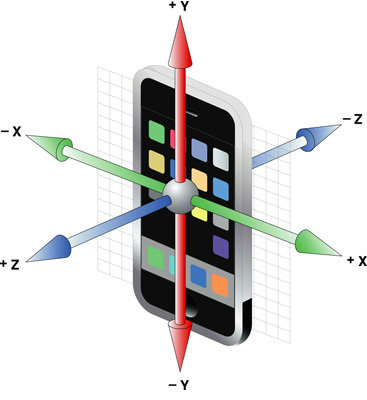Author Topic: Rotation/Acceleration -> Joystick values (with calibration)  (Read 1944 times)Kitty Hello

• code monkey
• Prof. Inline
••• Posts: 10713
• here on my island the sea says 'hello'Rotation/Acceleration -> Joystick values (with calibration)
« on: 2011-Oct-21 »
I did not solve it yet (did not have to  - but it's like this:)Code: GLBasic [Select]
WHILE TRUE
LOCAL gx,gy,gz
gx = GETJOYX(0)
gy = GETJOYY(0)
gz = GETJOYZ(0)

LOCAL angley = 90 + ATAN(gz,-gy)
LOCAL anglex = 90 + ATAN(gz, gx)

// print this angle now
PRINT INTEGER(anglex) + " / "+INTEGER(angley), 0,0
PRINT INTEGER(gx*100.) + " / "+INTEGER(gy*100.) + " / "+INTEGER(gz*100.), 0,20
SHOWSCREEN
WEND

So, now you have angles from the inclination. You can e.g. make that a joystick now by saying:

Joyx = max(-1.0, min(1.0, anglex / 30.0 ))

that way you take the angles from 0 to 30° as an axis amplitude of 0 - 1,0.
You can also make an "digital" stick that only returns 0 or 1 when you do:

Joyx = anglex / 5 // need 5 ° rotation to "press left/right"
if Joyx < 1 then Joyx = 0 // ignore too small rotations
Joyx = sng(Joyx) // now make it digital -1, 0, 1

Now for the calibration: In your "Pause" routine, calculate the "anglex, angley" values for the "silent" position. Then just subtract these values after the atan() lines.